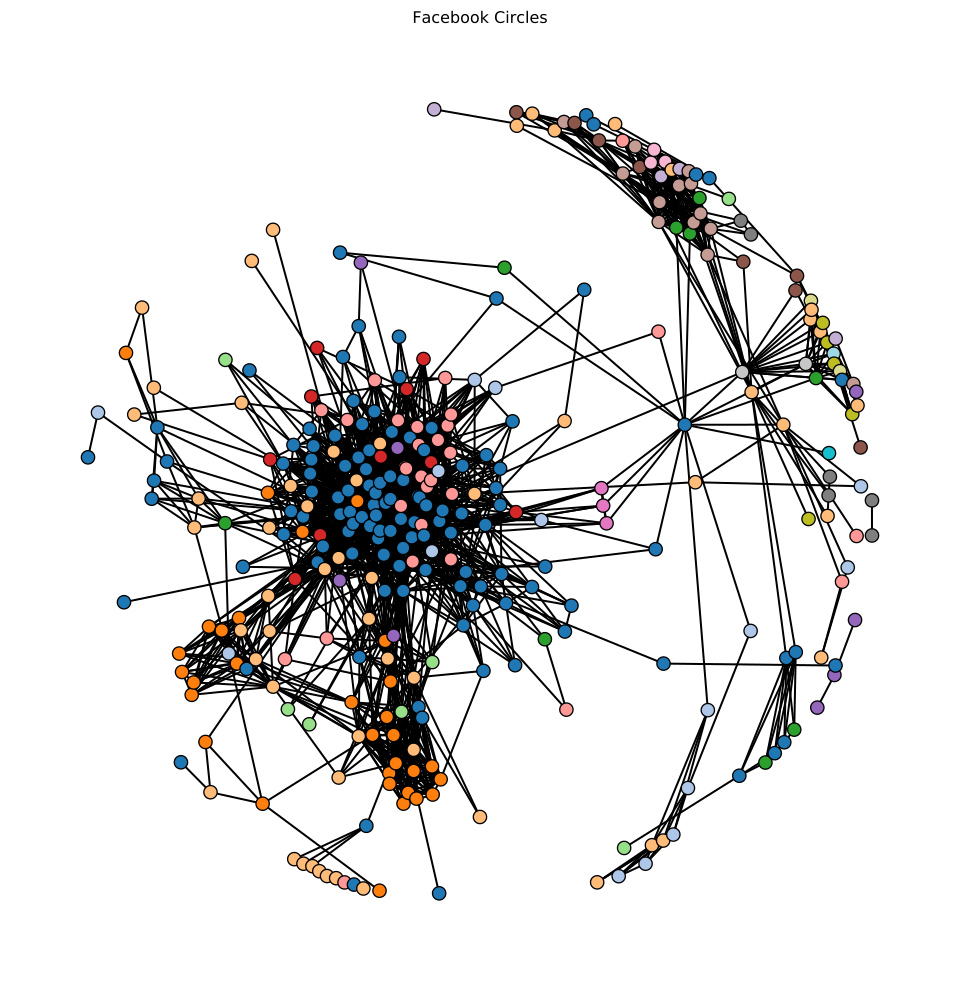Network graph¶

The data in this example represents Facebook social circle obtained from SNAP.

Most examples work across multiple plotting backends, this example is also available for:

In :
import pandas as pd
import holoviews as hv
hv.extension('matplotlib')
hv.output(fig='svg')Declaring data¶

In :

fb_nodes = hv.Nodes(nodes_df).sort()
fb_graph = hv.Graph((edges_df, fb_nodes), label='Facebook Circles')

Plot¶

In :
fb_graph.opts(
cmap='tab20', node_color='circle', fig_size=350, padding=0.1,
show_frame=False, xaxis=None, yaxis=None, node_size=10)
Out: